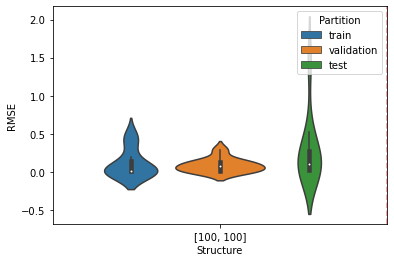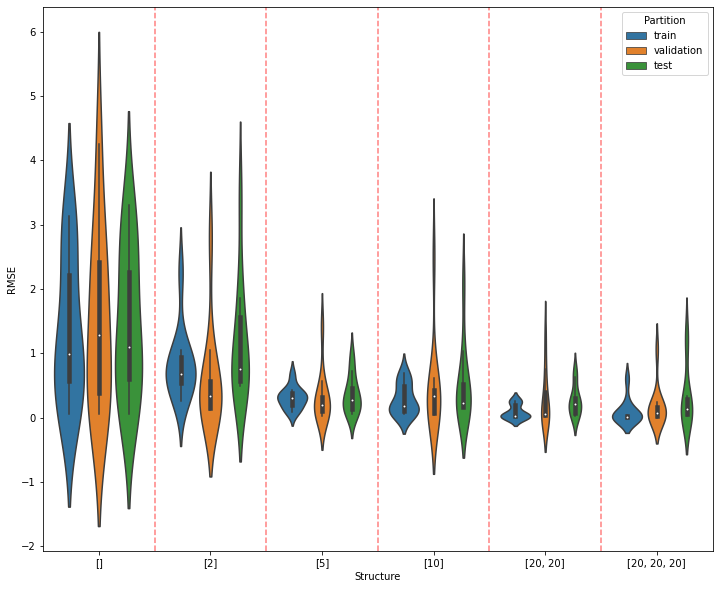Partitional Data to Compare Neural Network Performance

To fairly compare the performance of machine learning models, we must partition data into training, validation and testing parts. Remember, "partition" means disjoint subsets, ones whose intersection is empty. We will train our model on the training partition, calculate its performance every epoch on the validation partition, return the model with parameter values that resulted in the best performance on the validation partition, then apply the trained model on the testing partition and report its performance. It is only this last calculation, performance on the testing data, that is useful for predicting how well our model will do on new data.

One way to partition data info training, validation, and testing partitions is to simply randomly select samples from the available data into these three disjoint subsets. We can specify the size of each subset as fractions of the number of data samples.

It would be nice to have such a function, which could be called like this:

Xtrain, Ttrain, Xval, Tval, Xtest, Ttest = partition(X, T, fractions=(0.6, 0.2, 0.2),
shuffle=True, classification=True)


This would randomly take 60 % of the samples as training data, 20 % as validation data, and 20 % as testing data.

In fact, this would be so nice to have ... here it is!

In :
import numpy as np
import matplotlib.pyplot as plt

In :
def partition(X, T, fractions, shuffle=True, classification=False):
"""Usage: Xtrain,Train,Xvalidate,Tvalidate,Xtest,Ttest = partition(X,T,(0.6,0.2,0.2),classification=True)
X is nSamples x nFeatures.
fractions can have just two values, for partitioning into train and test only
If classification=True, T is target class as integer. Data partitioned
according to class proportions.
"""
train_fraction = fractions
if len(fractions) == 2:
# Skip the validation step
validate_fraction = 0
test_fraction = fractions
else:
validate_fraction = fractions
test_fraction = fractions

row_indices = np.arange(X.shape)
if shuffle:
np.random.shuffle(row_indices)

if not classification:
# regression, so do not partition according to targets.
n = X.shape
n_train = round(train_fraction * n)
n_validate = round(validate_fraction * n)
n_test = round(test_fraction * n)
if n_train + n_validate + n_test > n:
n_test = n - n_train - n_validate
Xtrain = X[row_indices[:n_train], :]
Ttrain = T[row_indices[:n_train], :]
if n_validate > 0:
Xvalidate = X[row_indices[n_train:n_train + n_validate], :]
Tvalidate = T[row_indices[n_train:n_train + n_validate], :]
Xtest = X[row_indices[n_train + n_validate:n_train + n_validate + n_test], :]
Ttest = T[row_indices[n_train + n_validate:n_train + n_validate + n_test], :]

else:
# classifying, so partition data according to target class
classes = np.unique(T)
train_indices = []
validate_indices = []
test_indices = []
for c in classes:
# row indices for class c
rows_this_class = np.where(T[row_indices,:] == c)
# collect row indices for class c for each partition
n = len(rows_this_class)
n_train = round(train_fraction * n)
n_validate = round(validate_fraction * n)
n_test = round(test_fraction * n)
if n_train + n_validate + n_test > n:
n_test = n - n_train - n_validate
train_indices += row_indices[rows_this_class[:n_train]].tolist()
if n_validate > 0:
validate_indices += row_indices[rows_this_class[n_train:n_train + n_validate]].tolist()
test_indices += row_indices[rows_this_class[n_train + n_validate:n_train + n_validate + n_test]].tolist()
Xtrain = X[train_indices, :]
Ttrain = T[train_indices, :]
if n_validate > 0:
Xvalidate = X[validate_indices, :]
Tvalidate = T[validate_indices, :]
Xtest = X[test_indices, :]
Ttest = T[test_indices, :]
if n_validate > 0:
return Xtrain, Ttrain, Xvalidate, Tvalidate, Xtest, Ttest
else:
return Xtrain, Ttrain, Xtest, Ttest

In :
X = np.arange(10).reshape(-1, 1)
T = X + 0.1
X, T

Out:
(array([,
,
,
,
,
,
,
,
,
]),
array([[0.1],
[1.1],
[2.1],
[3.1],
[4.1],
[5.1],
[6.1],
[7.1],
[8.1],
[9.1]]))
In :
def print_them(Xtrain, Ttrain, Xval, Tval, Xtest, Ttest):
print('Train')
print(np.hstack((Xtrain, Ttrain)))
print('Val')
print(np.hstack((Xval, Tval)))
print('Test')
print(np.hstack((Xtest, Ttest)))


Run the following cell several times to see different partitions.

In :
Xtrain, Ttrain, Xval, Tval, Xtest, Ttest = partition(X, T, (0.6, 0.2, 0.2), shuffle=True)
print_them(Xtrain, Ttrain, Xval, Tval, Xtest, Ttest)

Train
[[4.  4.1]
[8.  8.1]
[9.  9.1]
[0.  0.1]
[6.  6.1]
[2.  2.1]]
Val
[[5.  5.1]
[1.  1.1]]
Test
[[3.  3.1]
[7.  7.1]]


Now that we can partition as many times as we like, let's work on another function that returns the training performance, validation performance, and testing performance for multiple partitions.

But first, we need some data to play with.

In :
import pandas as pd
import os

if os.path.isfile('automobile.csv'):
else:
usecols=range(8))
automobile.columns = ('mpg', 'cylinders', 'displacement', 'horsepower', 'weight',
'acceleration', 'year', 'origin')

print(f'Number rows in original data file {len(automobile)}.')
automobile = automobile.dropna(axis=0)
print(f'Number rows after dropping rows with missing values {len(automobile)}.')

automobile.to_csv('automobile.csv', index=False)  # so row numbers are not written to file

Reading data from 'automobile.csv'.

In :
automobile

Out:
mpg cylinders displacement horsepower weight acceleration year origin
0 18.0 8 307.0 130.0 3504.0 12.0 70 1
1 15.0 8 350.0 165.0 3693.0 11.5 70 1
2 18.0 8 318.0 150.0 3436.0 11.0 70 1
3 16.0 8 304.0 150.0 3433.0 12.0 70 1
4 17.0 8 302.0 140.0 3449.0 10.5 70 1
... ... ... ... ... ... ... ... ...
387 27.0 4 140.0 86.0 2790.0 15.6 82 1
388 44.0 4 97.0 52.0 2130.0 24.6 82 2
389 32.0 4 135.0 84.0 2295.0 11.6 82 1
390 28.0 4 120.0 79.0 2625.0 18.6 82 1
391 31.0 4 119.0 82.0 2720.0 19.4 82 1

392 rows × 8 columns

Now we need a function that creates and trains a new neural network for each partition, and returns the results.

In :
def multiple_runs_regression(n_partitions, X, T, fractions, n_hiddens_list, n_epochs, learning_rate):

def rmse(Y, T):
return np.sqrt(np.mean((T - Y) ** 2))

print(f'Structure {n_hiddens_list}: Repetition', end=' ')
results = []
for rep in range(n_partitions):

print(f'{rep + 1}', end=' ')

Xtrain, Ttrain, Xval, Tval, Xtest, Ttest = partition(X, T, fractions,
shuffle=True, classification=False)

nnet = NeuralNetworkTorch(X.shape, n_hiddens_list, T.shape)
nnet.train(Xtrain, Ttrain, n_epochs, learning_rate, method='adam', Xval=Xval, Tval=Tval, verbose=False)

Ytrain = nnet.use(Xtrain)
Yval = nnet.use(Xval)
Ytest = nnet.use(Xtest)

structure = str(n_hiddens_list)
results.extend([[structure, 'train', rmse(Ytrain, Ttrain)],
[structure, 'validation', rmse(Yval, Tval)],
[structure, 'test', rmse(Ytest, Ttest)]])
print()
return results


Let's use the NeuralNetworkTorch that we defined in Lecture Notes 16.1. You will use that code in your solution to A5.

In :
from A5mysolution import *

In :
fractions = (0.6, 0.2, 0.2)
n_hiddens_list = [100, 100]
n_epochs = 1000
learning_rate = 0.001

n_partitions = 10
results = multiple_runs_regression(n_partitions, X, T, fractions, n_hiddens_list, n_epochs, learning_rate)

Hiddens [100, 100]: Repetition 1 2 3 4 5 6 7 8 9 10

In :
results

Out:
[['[100, 100]', 'train', 0.004062758725166755],
['[100, 100]', 'validation', 0.1353611102136738],
['[100, 100]', 'test', 0.030880251632869154],
['[100, 100]', 'train', 0.00823247983172531],
['[100, 100]', 'validation', 0.11043832114743887],
['[100, 100]', 'test', 0.028416360071150438],
['[100, 100]', 'train', 0.40122134940580084],
['[100, 100]', 'validation', 0.05924082369573957],
['[100, 100]', 'test', 0.23956245872430842],
['[100, 100]', 'train', 0.1901793548782251],
['[100, 100]', 'validation', 0.0063642058693349065],
['[100, 100]', 'test', 0.5241358454319545],
['[100, 100]', 'train', 0.008299690420836031],
['[100, 100]', 'validation', 0.08765151014254802],
['[100, 100]', 'test', 0.29181913369227463],
['[100, 100]', 'train', 0.014493253494874988],
['[100, 100]', 'validation', 0.00300954120162004],
['[100, 100]', 'test', 0.014333701341829257],
['[100, 100]', 'train', 0.0034862982859318057],
['[100, 100]', 'validation', 0.04208833444664035],
['[100, 100]', 'test', 0.11987970658415248],
['[100, 100]', 'train', 0.010900611299816763],
['[100, 100]', 'validation', 0.15167900315897329],
['[100, 100]', 'test', 0.025156286379082154],
['[100, 100]', 'train', 0.011939286853332511],
['[100, 100]', 'validation', 0.002145348144511191],
['[100, 100]', 'test', 0.0982790440174706],
['[100, 100]', 'train', 0.4793987304516573],
['[100, 100]', 'validation', 0.2912593675536652],
['[100, 100]', 'test', 1.4765085996927851]]

The above form of the results seems a little wordy, with the network structure and the words 'train', 'validation' and 'test' repeated so often. But you will soon see why I chose this form.

In :
resultsdf = pd.DataFrame(results, columns=('Structure', 'Partition', 'RMSE'))
resultsdf

Out:
Structure Partition RMSE
0 [] train 2.919725
1 [] validation 0.894220
2 [] test 2.252018
3 [] train 1.158353
4 [] validation 2.631544
... ... ... ...
175 [20, 20, 20] validation 0.184229
176 [20, 20, 20] test 0.045723
177 [20, 20, 20] train 0.008183
178 [20, 20, 20] validation 0.041767
179 [20, 20, 20] test 0.102511

180 rows × 3 columns

Violin plots can be a helpful way to visualize the distribution of values from multiple runs. An illustration of how a violin plot reveals more than a boxplot is shown at this site. Matplotlib includes a violinplot function documented here.

This example includes a link to a jupyter notebook. We can make a legend and x-axis labels as shown in the example at that site. We will use the seaborn plotting package version of violinplot. Another example is provided here.

Here is a simple example of how to make a violin plot showing train, validation, and test set error distributions for different hidden layer structures.

In :
import seaborn as sns

sns.violinplot(x='Structure', y='RMSE', hue='Partition', data=resultsdf)
for x in range(len(n_hiddens_list) - 1):
plt.axvline(x + 0.5, color='r', linestyle='--', alpha=0.5)To compare the performance of different neural networks, just wrap the call to multiple_runs_regression in a for loop over different structures and collect the results.

In :
fractions = (0.6, 0.2, 0.2)
n_hiddens_list = [[], , , , [20, 20], [20, 20, 20]]  # Notice the first one... []
n_epochs = 1000
learning_rate = 0.001

n_partitions = 10

results = []
for nh in n_hiddens_list:
results.extend(multiple_runs_regression(n_partitions, X, T, fractions, nh, n_epochs, learning_rate))

resultsdf = pd.DataFrame(results, columns=('Structure', 'Partition', 'RMSE'))

Hiddens []: Repetition 1 2 3 4 5 6 7 8 9 10
Hiddens : Repetition 1 2 3 4 5 6 7 8 9 10
Hiddens : Repetition 1 2 3 4 5 6 7 8 9 10
Hiddens : Repetition 1 2 3 4 5 6 7 8 9 10
Hiddens [20, 20]: Repetition 1 2 3 4 5 6 7 8 9 10
Hiddens [20, 20, 20]: Repetition 1 2 3 4 5 6 7 8 9 10

In :
resultsdf

Out:
Structure Partition RMSE
0 [] train 2.919725
1 [] validation 0.894220
2 [] test 2.252018
3 [] train 1.158353
4 [] validation 2.631544
... ... ... ...
175 [20, 20, 20] validation 0.184229
176 [20, 20, 20] test 0.045723
177 [20, 20, 20] train 0.008183
178 [20, 20, 20] validation 0.041767
179 [20, 20, 20] test 0.102511

180 rows × 3 columns

In :
plt.figure(figsize=(12, 10))
sns.violinplot(x='Structure', y='RMSE', hue='Partition', data=resultsdf)
for x in range(len(n_hiddens_list) - 1):
plt.axvline(x + 0.5, color='r', linestyle='--', alpha=0.5)